Journal DetailsFormat
Journal
eISSN
2083-134X
First Published
16 Apr 2011
Publication timeframe
4 times per year
Languages
English
Open Access

# Finite element modeling of continuous drive friction welding of Al6061 alloy

###### Accepted: 21 Feb 2021
Journal DetailsFormat
Journal
eISSN
2083-134X
First Published
16 Apr 2011
Publication timeframe
4 times per year
Languages
English

Continuous drive friction welding process is widely used in various industrial applications to assemble shafts, tubes, and many other components. This paper's motivation was developing a CDFW model using the Finite Element Method (FEM). The coupling of the process's thermal and mechanical behaviors was considered during the simulation by COMSOL Multiphysics®. The construction of phase transition curves for Al6061 allowed determining several temperature-dependent thermophysical properties of the material. These properties are then injected in a second simulation to study the temperature evolution during welding. Subsequently, these results are compared and analyzed with the experimental outcomes. Excellent comparability between the model and experimental results was achieved. A unique phenomenon in the welding temperature profile was observed and explained through the model and experimental results interpretation.

#### Keywords

Introduction

Friction welding is the operation of joining metallic parts in the solid phase. Unlike conventional welding mechanisms, friction welding provides a perfect joint without reaching the material's melting temperature. This original technique preserves the energy required for welding, the properties of the materials, and prevents, under specific conditions, the formation of intermetallic layers. Moreover, friction welding offers substantial improvements in the speed of the process, welding of various types of materials and geometries, environment friendliness, and the ability to weld similar and dissimilar materials.

Welding metal parts via friction welding can be summarized into three general types; friction stir welding, rotary friction welding (RFW), and friction stir spot welding. Each of these techniques is unique regarding the application and the welding procedure. RFW, in general, as presented in Figure 1, is based on the idea of rubbing two parts (by rotation) against each other, causing friction and heat generation. As a result, joining the two pieces while remaining in the solid-state phase. One part is usually attached to a rotating mechanism; the rotation is applied and controlled from this side. The other part is kept fixed (non-rotating), and an axial force is applied to this part. Two main types of rotary friction welding can be distinguished: continuous drive friction welding (CDFW) and inertia friction welding (IFW).

In CDFW, the rotation speed is maintained for a specified friction time while applying a friction pressure through the axial force. The rotation is then stopped, and a higher forging (upset) pressure is applied for a predetermined forging (upset) time. As for IFW, the rotating part is disengaged (not stopped), then the pressure is applied, and the friction process occurs until the rotating part comes to a complete stop. A higher upset (forge) pressure can be applied afterward for a specific upset (forging) time. The main difference between CDFW and IFW is that the rotation speed in CDFW is kept constant for the friction time duration, whereas the rotation speed in IFW is changing (decreasing) throughout the friction time. [1, 2]

It should be noted that the force and the rotation applied must follow temporal variations that are well defined. These variations are generally predetermined for CDFW, as shown in Figure 2. These variations specify the process parameters. Constant rotational speed and constant friction pressure are maintained for a predefined friction time and followed by a higher forging pressure for a shorter upset time with no rotation. The temperature rises because of the friction forces on the contact zone; the friction pressure during the process causes the two parts to be welded together. CDFW is widely used to assemble shafts, tubes, and many geometries of many industrial applications. For example, this welding process is used in many automotive parts such as cluster gears, rotor assemblies, and compressors. This welding method is also used in the oil and gas industry to produce various components such as valve bodies, core drills, and oil well drills.

In general, mathematical modeling [3, 4] of any process can provide means for the apprehension, the prediction of the attributes of the process, and its performances. The characteristics and process parameters of CDFW can be analyzed by mathematical modeling; thus, saving time and money. The consistency of the mathematical models of CDFW with the experimental results can be verified by comparing the temperature profile during the welding process for both methods. Verifying the Finite Element Method (FEM) outcomes with experimental results in temperature profiles have been used widely. A thermocouple is usually used during the experimental procedure; the temperature vs. time (T vs. t) profile is created, and the same output from the FE model is then compared.

Literature review

The model established by Li et al.  used the measured power method, assuming that the heat flux is evenly distributed over the contact interface. Experimental measurements were then used to validate the model. A similar approach was established by Kalsi and Sharma  and Nguyen . Maalekian [8, 9] published a comparative study of heat production and, later, the temperature prediction for steel's friction welding. The authors used DEFORM™ software to check results and temperature-dependent properties. Comparing the temperature profiles obtained was established with four different methods: the constant coefficient of friction method, the slip method, the power method, and the inverse method. The last two methods showed excellent results because they were based on experimental results. The models established by Can et al.  have produced similar results using mathematical modeling via Quick-Field® software. The results indicated the dependence of the temperature profiles on the welding parameters and sample sizes.

In another study, Maalekian  has reported that experimental data-based methods produce exceptional results, but the mathematical model profiles were not reliable. Indeed, the author confirmed this fact, and no improvement in the mathematical model results was observed. The studies of Özdemir And Bouarroudj et al. [12,13,14] were limited. For example, the temperature dependence was studied on the axial direction only (along the work-piece's outer radius).

The main limitation in most models presented in the literature is using a constant-temperature material property (density, specific heat, coefficient of thermal expansion, and conductivity) while modeling the welding process. The temperature independence can generate numerous errors in the model since these properties change as the material's phase change due to temperature variation. Also, many research efforts considering the FEM of CDFW are based on material properties independent of temperature change or based on a set of incomplete temperature-dependent material properties. To accurately perform FEM of CDFW, one should consider the continuous variations in the material properties as a function of temperature over the entire temperature range as the phase of the material changes within the solidus and liquidus regions of the phase diagram. Upon establishing that, the FEM can simulate CDFW accurately while considering both the heat generation aspect of the welding and the mechanics of solids aspect.

COMSOL Multiphysics® software library is very well established and contains most material properties given for a specific condition at room temperature. However, the software model building allows the user to determine and choose the material's temperature-dependent properties, define expressions or profiles for these properties, and incorporate these temperature-dependent properties in the FEM analysis. As a result, the material properties in the model instantaneously change depending on the model's conditions. Thus, such models should provide more robust and accurate outcomes since these properties vary in actual experiments depending on the process's instantaneous temperature.

This research aims to develop and verify a mathematical model that replicates the actual CDFW for Al6061. Also, this work aims to provide a substantial contribution to the development of CDWF modeling. This work's motivation is building a model that simulates the welding process considering the possible change of the physical properties with temperature. Modeling the process of welding while considering the temperature-dependent physical properties is not widely investigated in the literature. Such valid FE models can reduce the cost and the duration of experiments significantly.

First, a numerical approach is utilized to define the material's temperature-dependent thermo-physical properties, i.e., determine the material properties as the phase of the material transition from solid to liquid. Second, a numerical simulation of the CDFW model is established to obtain the welding temperature profiles incorporating the "heat transfer in solids" and the "mechanical structural" modules in COMSOL Multiphysics®, considering the thermo-physical properties defined earlier. The temperature profiles from the model and the experiments were used to validate the numerical simulation.

Materials and methods
Determination of thermo-physical proprieties of Al6061

Numerical simulation by COMSOL Multiphysics has been developed to study the phase transition of aluminum 6061 using apparent heat capacity and establishing a phase-change model for such material properties. This technique was used by Dawood et al.  for similar and dissimilar materials, considering the dependence of specific heat on temperature and not the other related material properties. Nevertheless, it allows estimating the phase change coefficient α(T) and subsequently determine the material properties as functions of temperature. The properties of interest are the heat capacity (Cp), as indicated in Equation 1, the density (ρ), as shown in Equation 2, and the thermal conductivity (κ), as shown in Equation 3.

$Cp(Jkg)=Cp,solid.(1−α(T))+Cp,liquid.α(T)+L1→2dαdT'$ {C_p}\left( {{J \over {kg}}} \right) = {C_{p,solid}}.\left( {1 - \alpha \left( T \right)} \right) + {C_{p,liquid}}.\alpha \left( T \right) + {L_{1 \to 2}}{{d\alpha } \over {d{T^\prime}}} $ρ(kgm3)=ρsolid.(1−α(T))+ρliquid.α(T),$ \rho ({{kg} \over {{m^3}}}) = {\rho _{solid}}.\left( {1 - \alpha \left( T \right)} \right) + {\rho _{liquid}}.\alpha \left( T \right), $k(Wm⋅K)=κsolid.(1−α(T))+κliquid.α(T),$ k\left( {{W \over {m \cdot K}}} \right) = {\kappa _{solid}}.\left( {1 - \alpha \left( T \right)} \right) + {\kappa _{liquid}}.\alpha \left( T \right),

Where, α(T) = 0 for a pure solid material and α(T) = 1 for a pure liquid material. The last term in equation 1 $(L1→2dαdT')$ ({{\rm{L}}_{1 \to 2}}{{{\rm{d}}\alpha } \over {{\rm{d}}{{\rm{T}}^\prime}}}) represents the total amount of latent heat in the process. Resulting from the multiplication of the term L1→2 representing the change in latent heat between the two phases and the term $dαdT'$ {{{\rm{d}}\alpha } \over {{\rm{d}}{{\rm{T}}^\prime}}} representing the integration of the phase change coefficient during the interval ΔT1→2.

The material properties change continuously in the transition range, i.e., as the temperature changes, the phase changes from solid to liquid and vice versa. Several material properties are required, gathered, and introduced in the phase change simulation to properly establish the aluminum 6061 phase-change model. These data are illustrated in the Table 1.

Al6061 material properties for phase-change simulation. 

Property Value
Ttrans [K] 925.15
δT [K] 50
Δhf [kJ/kg] 380
ρsolid [kg/m3] 2705
ρliquid [kg/m3] 2415
Cp,solid [J/kg·K] 870
Cp,liquid [J/kg·K] 1170
κsolidus [W/m·K] 66.5
κliquid [W/m·K] 90

Where, Ttrans is the transition temperature of Al6061 in (K), δT is the transition interval in (K), Δhf is the change in the latent heat capacity of the material in (kJ/kg), ρ is the density of the material in (kg/m3), Cp is the specific heat capacity in (J/kg·K), and κ is the thermal conductivity in (W/m·K). The thermal conductivity values indicated in Table 1 correspond to the conductivity of the material as it reaches the solidus line (κsolidus) and the conductivity of the material when melted (κliquid) [17, 18]. In the beginning, the thermophysical proprieties of these two phases of aluminum 6061 were added to the model. The heat transfer model was then adapted to reproduce the phase change of the metal. This model was based on a time-dependent numerical resolution of the following differential equations in respect to heat transfer and phase transition:

$ACρCp∂T∂t+ACρCpu∇T+∇.q=ACQ+q0+ACQp+ACQvd,$ {A_C}\rho {C_p}{{\partial T} \over {\partial t}} + {A_C}\rho {C_p}u\nabla T + \nabla .q = {A_C}Q + {q_0} + {A_C}{Q_p} + {A_C}{Q_{vd}}, $q=− ACk∇T,$ q = - \;{A_C}k\nabla T,
Numerical simulation of CDFW COMSOL

Multiphysics® uses the finite element method to extend conventional models for one type of physical phenomenon in Multiphysics models and solves coupled physical phenomena. This approach reproduces the CDFW by simultaneously solving heat transfer equations and mechanical deformation while respecting the interaction and the dependence between the two phenomena. The numerical simulation of CDFW consisted of defining the geometry, defining the coupled heat transfer and mechanical deformation models, defining boundary conditions, meshing the model, and determining the model solving conditions to run the model and generate results.

The first step of the numerical simulation was choosing the geometry. In this study, a 2D axisymmetric geometry, as shown in Figure 3, was adapted to minimize the number of iterations and reduce the simulation time. It also allows representing the simulation results as 3D shapes and plots.

The second step of the simulation was concerned with the appropriate choice of the correct models for CDFW. Several considerations must be taken into account to implement the friction effects in the contact zone between the two parts and the resulting increase of the temperature accurately, namely:

A complete definition of the metal's temperature-dependent properties, which is the results of the first simulation.

A coupled finite element model that incorporates both the mechanical deformation of the metal and heat transfer due to the friction process.

The mechanical deformation model considered the rotating aspect of the welding process and the friction pressure. The friction pressure was incorporated into the model as an axial force in transition and applied on the fixed part's outer surface (the fixed part's flat end). The model describing the mechanical behavior of the material in the welding process assumes a linearly elastic material governed by the following equations:

$∇S+Fv=0,$ \nabla S + {F_v} = 0, $ε=12((∇u)T+∇u)),$ \varepsilon= {1 \over 2}((\nabla u{)^T} + \nabla u)),

The term ∇S is the gradient of the total stresses within the volume in (N/m3), the term Fv is the force per unit volume (N/m3) applied in all directions. The strain equation indicated in Eq. 7 is a matrix representation in terms of the displacement gradient ∇u of the infinitesimal strain tensor ɛi,j expressed within the volume as: $εi,j=12(ui,j+uj,i),$ {\varepsilon_{i,j}} = {1 \over 2}({u_{i,j}} + {u_{j,i}}),

The heat transfer model suggested a heat source at the contact zone that is a function of the pressure (force per area), the rotational speed, and the part's characteristic dimension. It is a time-dependent model based on the following governing equations: $ρCp∂T∂t+ρCpu∇T+∇.q=Q,$ \rho {C_p}{{\partial T} \over {\partial t}} + \rho {C_p}u\nabla T + \nabla .q = Q, $q=−k∇T,$ q = - k\nabla T,

Where ρ is the density in (kg/m3), Cp is the specific heat capacity in (J/kg·K), u is the displacement in (m), ∇T is the temperature gradient in (K), ∇q is the gradient of the heat transfer rate in (W/m), Q is the total heat transfer in (W), and k is the thermal conductivity in (W/m·K).

Boundary conditions

The boundary conditions are set based on the model aspects being considered, as shown in Table 2. The solid mechanics model has the following boundary conditions: an axial symmetry, free to deform, zero initial displacements, rigid motion suppression on the fixed part, an axial force applied to the end of the fixed part, and a rotational motion applied on the rotating part. On the other hand, the heat transfer model had the following boundary conditions: an initial temperature condition being the same as the room temperature, axial symmetry, and a convection heat flux on the outer surfaces of the geometry due to the interaction of the model with the surroundings. The rotation and pressure conditions are controlled according to the actual friction time tf, as shown in Table 2.

FEM boundary conditions.

Model Feature Boundary Condition
Solid Mechanics The central axis of the parts Axial Symmetry
The outer surfaces of the parts Free to deform
The Entire model Zero initial displacement
The rotating part Rigid motion suppression
The end of the fixed part Axial load

Heat Transfer T = 305 K Initial temperature
The central axis of the parts Axial symmetry
The outer surfaces of the parts Heat flux according to q0 = h · (Text − T)

Welding Parameters Rotation 0 s to tf = rotation speed, otherwise zero
Pressure 0 s to tf = applied pressure, otherwise zero
Meshing the model

A subdivision of the mesh domain has been adopted to solve the established differential equations. The COMSOL Multiphysics® calculation code can use a square, a triangular, or other more complex elements. Using a non-regular subdivision on the studied system is widely used. The mesh can be narrowed near places of interest (in our case, the two-part contact zone), as shown in Figure 4(a).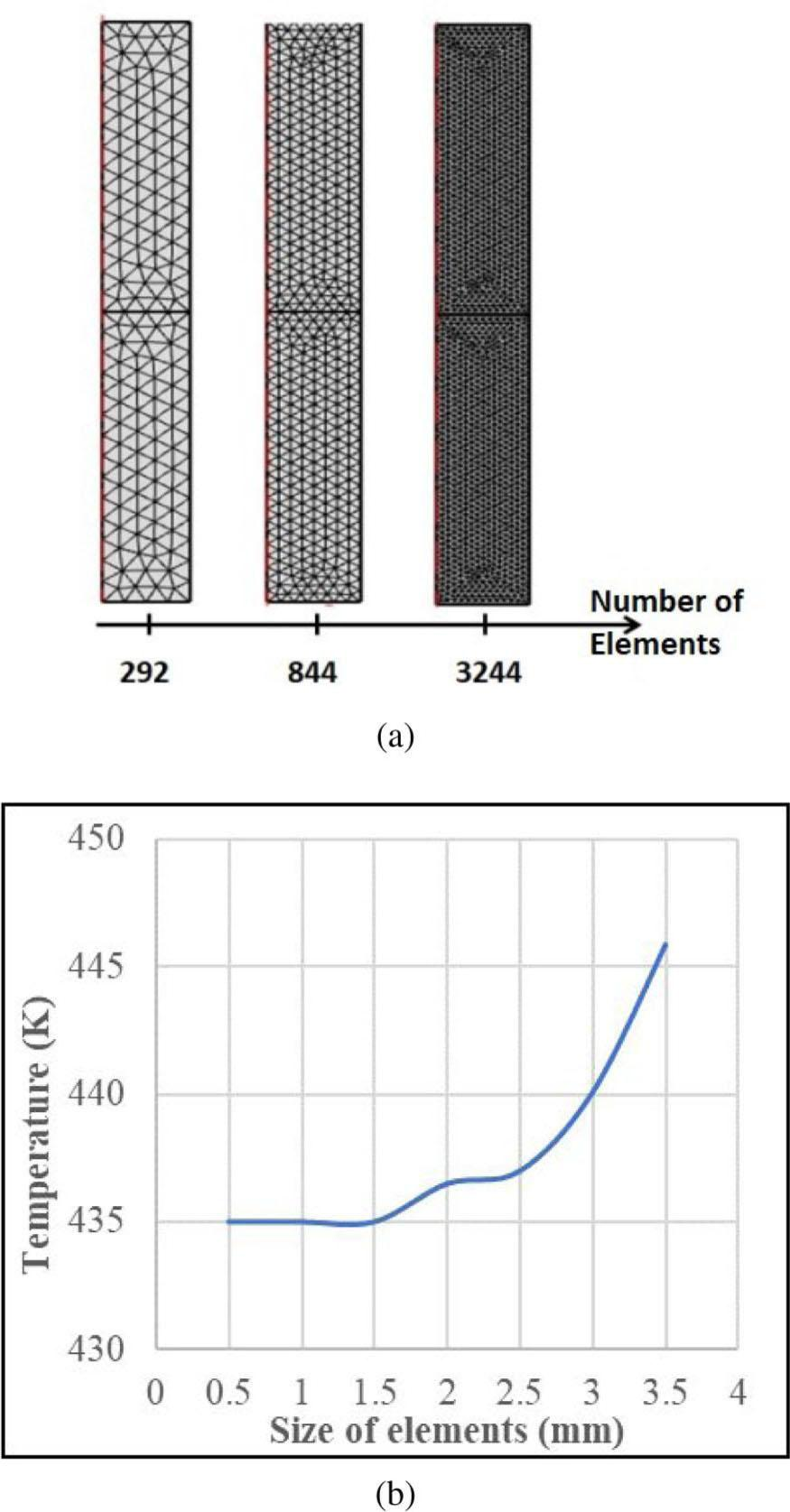a) the number of elements and fineness of the mesh and, b) convergence plot of the size of elements.

The calculation time and the precision of the results are directly related to the elements’ quality and size. Indeed, the accuracy of the results increases when the size of the elements decreases. The maximum number of elements chosen is limited by the free memory of the computing device and the time of numerical resolution. As such, a mesh convergence was performed, as shown in Figure 4(b), indicating the appropriate element size that provides acceptable results without exhausting the computational resources. It seems that element sizes about 1.5 mm and smaller resulted in repeatable modeling outcomes. The calculation time for such element sizes was reasonable and was around one hour.

Experimental procedure
Welding procedure

Verifying the model's output requires a series of experiments. The welding was accomplished using a conventional lathe machine. The rotating side was attached to the chuck of the lathe. While the fixed part was attached to a simple spring assembly that was fixated on the lathe machine. A centering apparatus was used to assure that the fixed part position is maintained and that no vibrations would occur on the fixed side. The two parts are then brought together until contact is achieved. The spring was then compressed according to the specified force used for welding. The spring compression was verified on the lathe machine and maintained during the welding procedure for the required welding duration. Once the welding duration is reached, the force application was adjusted accordingly, either increased to apply more force for the forging process or removed for cases where no forging was applied. Various trial and error runs were conducted to assess the need for forging and obtain operation boundaries. These preliminary experiments outlined the welding temperature profile with and without forging. The results indicated that forging was not required (no significant difference in the temperature profile), and thus, no forging was applied for later experiments.

Materials

The material used in the welding process was aluminum 6061. The chemical composition in weight percent of Al6061 is shown in Table 3. The two parts to be welded were machined to the final welding dimensions, as shown in Figure 5. There is a difference in the dimensions of the two parts to be welded. This difference arose because the small-diameter portion of the fixed part needed to be maintained for a long distance to prevent the sample's base from colliding with the centering apparatus (Figure 5, right side). The same length was used for the rotating side first; it generated high vibrations and unreliable temperature data. As a result, the length of the small-diameter portion of the rotating side was kept as short as possible to eliminate vibrations and obtain more accurate temperature profiles (Figure 5, left side). The geometry of the model was also adjusted accordingly. The welding process parameters used in this experiment are shown in Table 4.

Chemical composition in weight percent of Al6061.

Element Si Fe Cu Mn Mg Zn Cr Ti Al
Wt% 0.75 0.5 0.5 0.15 0.9 0.03 0.03 0.05 Rest

CDFW process parameters.

Process Parameter Value / range
Rotational Speed (rpm) 2000
Friction Pressure (MPa) 21
Friction time (s) 4, 5, 6, 9, 10, 12
Temperature measurement

A dual laser pointer infrared thermometer was used to monitor the welding temperature's evolution at the outer edge of the contact surfaces to be welded; this point will be referred to as point X. The laser pointer had an operational range of −50 to 800 °C, a spatial accuracy of 1 mm, and time accuracy of 0.5 s. The laser pointer was calibrated before being used in the welding process. The following welding times were used: 4, 5, 6, 9, 10, and 12s. The laser pointer screen's temperature data was video recorded and then analyzed using a computer for each case. Thus, it allowed developing experimental temperature versus time curves for each welding time considered. These curves were then compared to the Comsol Multiphysics® model output under similar conditions.

Results
FEM

As mentioned earlier, the phase transition model was used to obtain temperature-dependent material properties, and then these properties were implemented in the FE model of CDFW. Once the phase change corresponding to temperature was verified, all the temperature-dependent material properties can be identified. These properties are specific heat, density, and thermal conductivity. The coupling of the heat transfer and mechanical deformation phenomena becomes more meaningful since material properties become temperature-dependent. Thus, the thermal gradient within the welding zone and the temperature evolution can be displayed and analyzed as a welding time function.

Phase change model

The simulation results shown in Figure 6 predict that aluminum 6061 starts transitioning into a liquid phase at a temperature of 900 K. A zone of coexistence of the solid and liquid phases between 900 and 950 K is maintained. From 950 K upwards, all the aluminum is transformed into the liquid phase.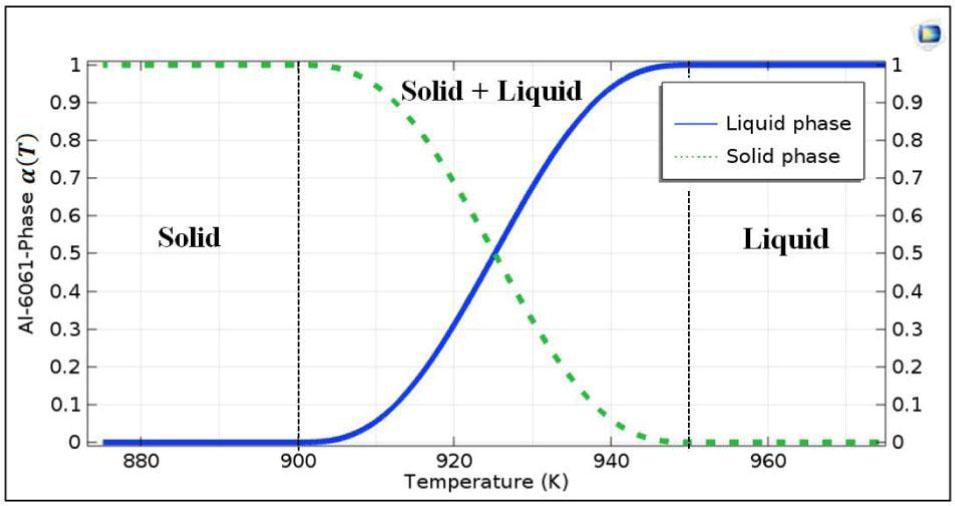Variation of the phase change coefficient as a function of the temperature of aluminum 6061.

This variation of the phase as a function of temperature, as shown in Figure 6, made it possible, by applying equations 1, 2, and 3, to plot the thermo-physical properties of the metal under study. Figures 7, 8, and 9 show the evolution of the specific heat capacity, the density, and the thermal conductivity of the aluminum 6061 based on the phase change model established, respectively. These temperature-dependent material properties were then used as functions defining the material properly in the FE model of CDFW to simulate the material behavior during the welding process precisely.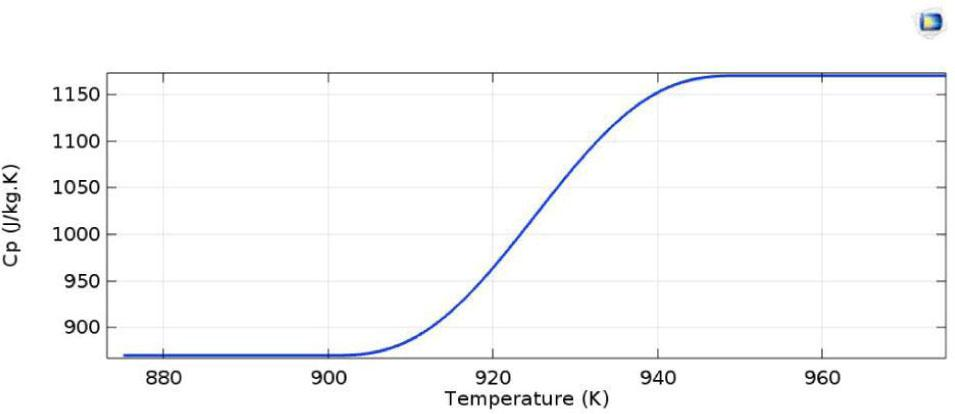Variation in the specific heat capacity of AA6061 as a function of temperature.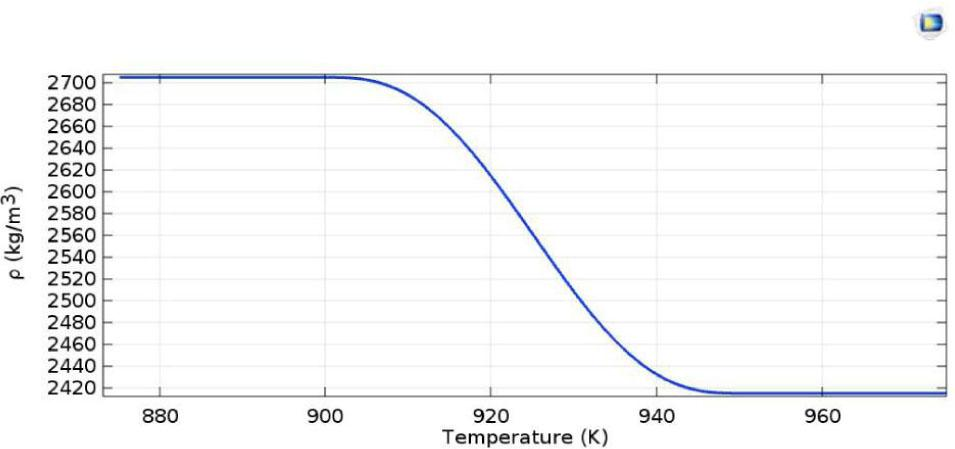Variation in the density of AA6061 as a function of temperature.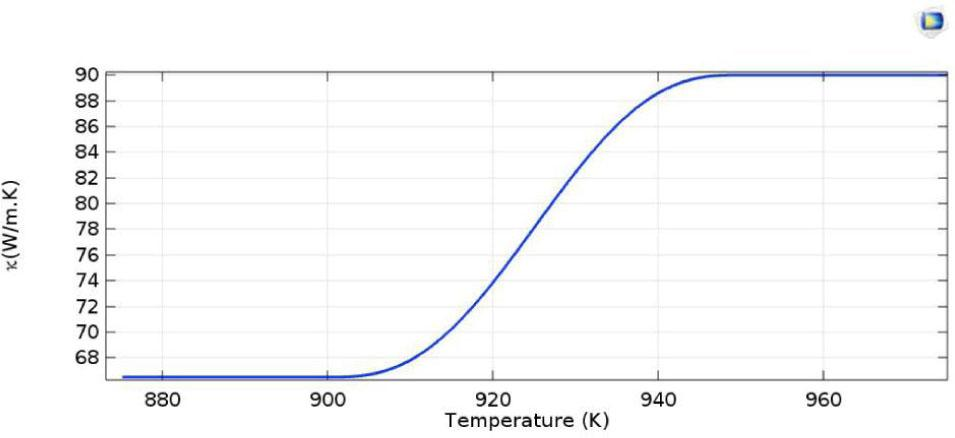Variation in the thermal conductivity of AA6061 as a function of temperature.
CDFW parameters

Figure 10 shows the welding parameters as a function of time as generated from the model. These are the same CDFW parameters that were used in the experiments for the 10 s friction time. These parameters are a rotational speed (red-dashed line) of 2000 rpm and a friction pressure (green-dashed line) of 21 MPa. As mentioned above, no upset was considered, and hence the upset pressure was zero, and consequently, the upset time was zero. Also, the 10 s friction time was used to model the temperature profiles around the welded surfaces as a function of time.

Validation of the model

The FEM model was verified using the temperature as a function of time obtained experimentally and from the model. This method of validating the FEM was implemented by Dawood et al.  and proved to be a suitable model validation method. A series of welding times, as mentioned above, were considered, namely, 4, 5, 6, 9, and 10 s. The data was collected from both sources and plotted in an excel sheet. The reason behind choosing these welding times was to observe the formation of flash as a function of the welding time for the material and explain a phenomenon observed for longer welding times. All experimental temperature measurements were obtained at point "X" defined earlier.

At first, longer welding times were considered. Some trial and error samples were made to smooth out the welding procedure, ensure all experiments are repeated simultaneously, and minimize any error source. So, a friction time of 12 s was chosen. Three replicas of the same welding conditions were fabricated to verify the experiment-model agreement. Temperature data were recorded in all three samples, compiled in Excel®, and plotted as an average experimental temperature profile. Figure 11 compares the average experimental temperature profile to that obtained from the model.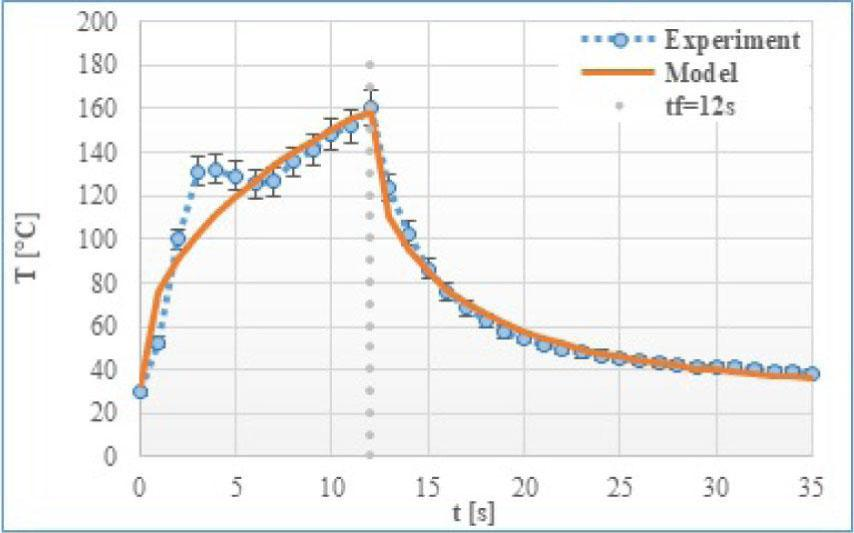The temperature profile for the 12 s friction time.

As shown in Figure 11, the model is in good agreement with the experiment. Both methods reached a peak welding temperature of 160 °C. Two distinct features were observed from the two curves. The first is the discrepancy between the experimental and model curves during the friction time, i.e., from zero to 12 s. The experimental curve exceeds the model curve at ∼ 3 s, reaches a notable increase at ∼ 135 °C, then falls back gradually until 6 s to ∼ 125 °C, after which it coincides with the model curve until the end of the friction time at 12 s. This phenomenon was not observed/discussed in previous studies. The second feature is the cooling curves, there seems to be a slight difference between the two curves, but generally, both cooling portions of the curves are almost identical. This figure showed good agreement between the experimental and model outcomes and provided confidence in the model, which allowed exploring various welding times.

Figure 12 compares the experimental to model temperature profiles for the friction times of 4, 5, and 6 s. The agreement in the maximum temperature between the experimental and model curves for the 4 s friction time is evident. On the other hand, comparing the 5 and 6 s curves, there seems to be some difference in the maximum welding temperature in both figures, but this difference does not exceed 10% and hence is acceptable. Also, there seem to be some differences between the experimental and model curves before reaching the peak temperature for all three friction times. For some reason, the experimental reading appears to be much less than the model reading. Although the difference is noticeable, it can be neglected since the peak temperature is in good agreement corresponding to previous studies . Moreover, a difference between the curves in terms of the number of peaks starts to become distinguishable. At 4 s, one temperature peak was observed, whereas two peaks were observed at 5 s. This effect is more clearly detected and somehow enlarged in the experimental curves for longer friction times, as shown in Figure 12 for 6 s and in Figure 13 for 9 and 10 s.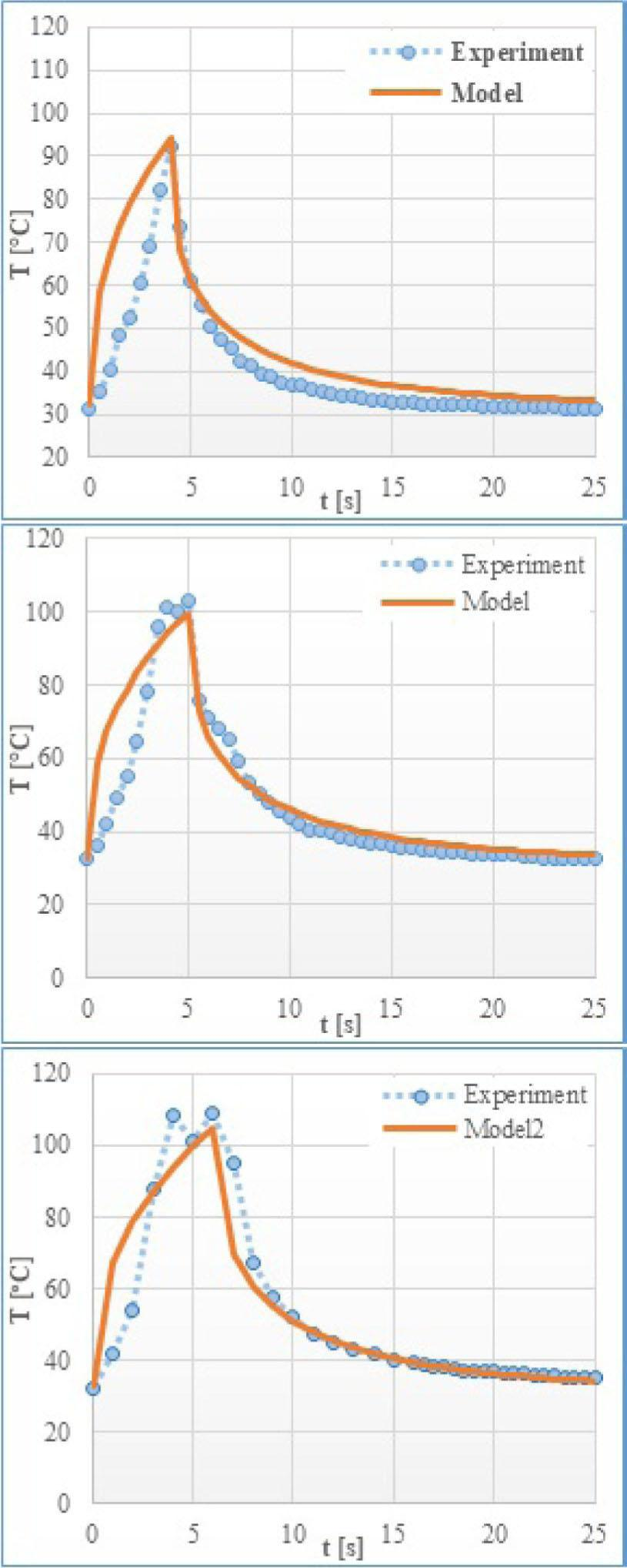Temperature profiles for different friction times; 4, 5, and 6 s, respectively.

Considering the higher friction times curves, Figure 13 shows the comparison between the experimental and model curves at 9 s and 10 s, respectively. As before, the peak temperature is in excellent agreement between the two methods for both welding times. The variation among the experimental and model curves in the time interval of 4 to 6 s in these figures is evident.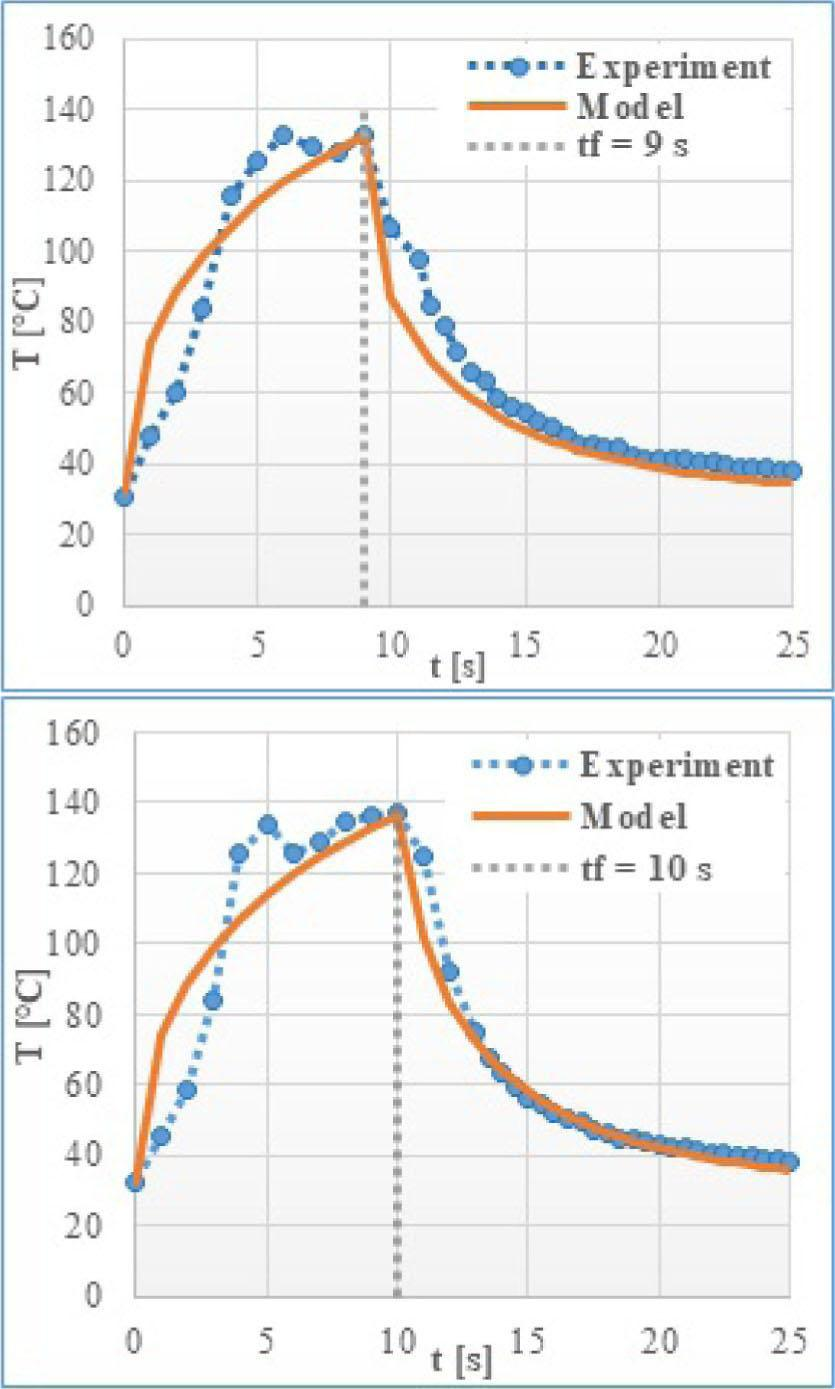The temperature profile for 9 and 10 s friction times.

In general, it seems that the experimental temperature measuring method implemented provided an excellent agreement when it comes to the maximum temperature. However, unexpected temperature profiles within the curves for friction times longer than 4 s were observed. The extent of this occurrence depends on the welding time used and usually lasts for about 2–3 s before providing similar readings to what the model provided. This experimental reading issue turns out to be related to the temperature measurement process and the flash evolution during the actual welding.

The series of welding times considered revealed that the flash starts to form in this type of material just after 4 s. The nature of the formation of the flash being a plasticized material moving outwards supports this idea. What was observed in the experimental temperature profiles as inconsistencies to the model temperature profiles is attributed to not measuring the temperature at the same point in both methods. In other words, as the flash grows, the point at which the experimental reading was measured is a little bit different from the original point X indicated earlier. As the flash is later completely formed at about 6–7 s, the experimental reading location goes back again very close to the original point. Hence, the agreement in the curves beyond 6 or 7 s. Figure 14 shows a comparison of the welding flash formation for different welding times. It indicates the absence of the flash at 4 s, its evolution at 5 and 6 s, and its final shape at 9 and 10 s. These figures clearly explain the differences between the experimental and model comparisons shown and indicated earlier.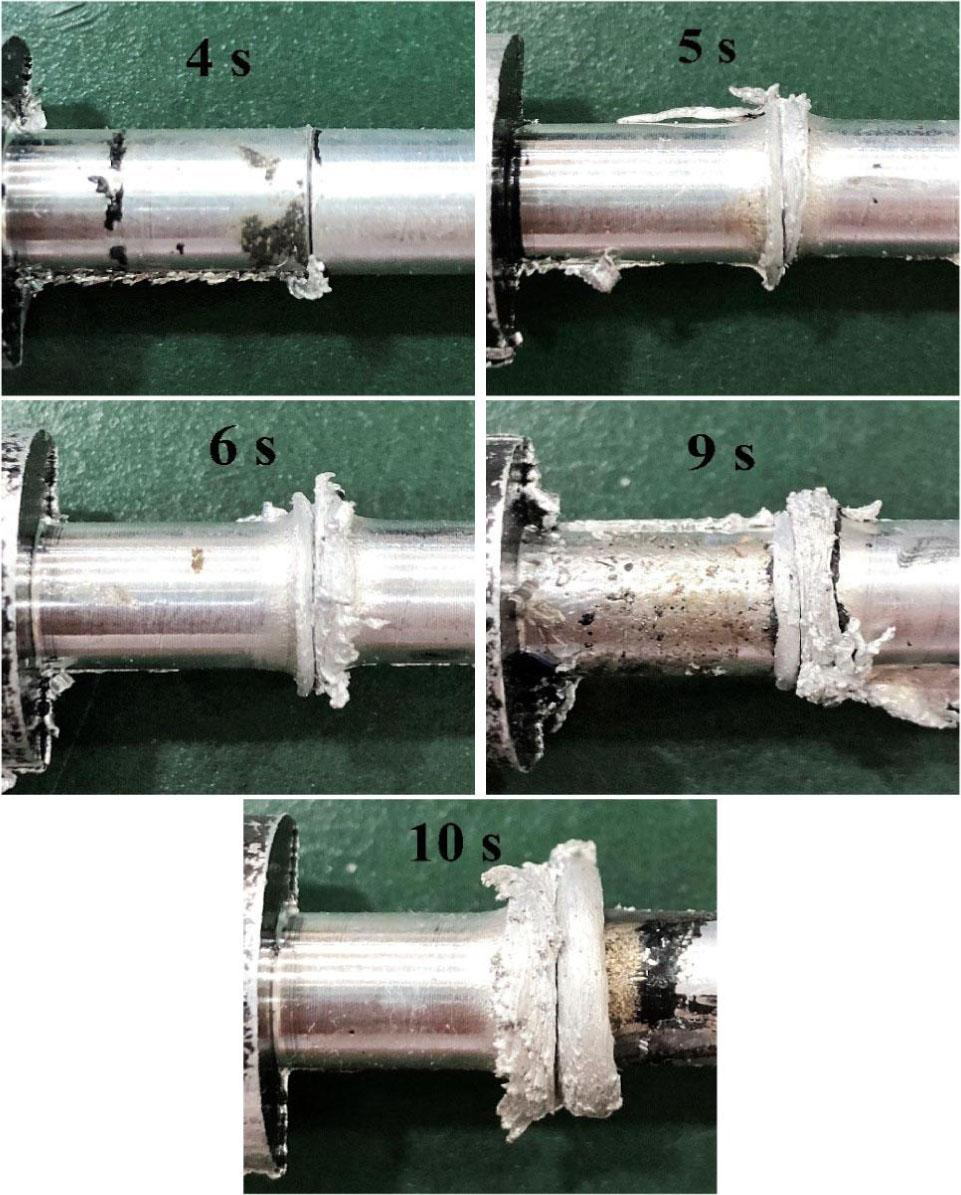Welding flash formation at different friction times; these are 4, 5, 6, 9, and 10 s, respectively.
Temperature profile

The temperature profile at the contact surface as time steps progressed from the beginning of the welding procedure until the end is one of these beneficial outcomes of FEM. Visualizing the temperature profile as 3D images for such time steps can help obtain some information related to the heat affected zone HAZ of the weld. Among the determining factors in the quality of friction welding are the flash and the HAZ . It is desirable to control the welding process parameters such that it produces the least amount of flash and as uniform as possible HAZ. The temperature profiles at specific time steps (t = 0, 1, 5, 10, and 11 s) are shown in Figures 15 to 18. These thermal profiles provide information about the HAZ development as the welding progresses from the start to the end of the cycle.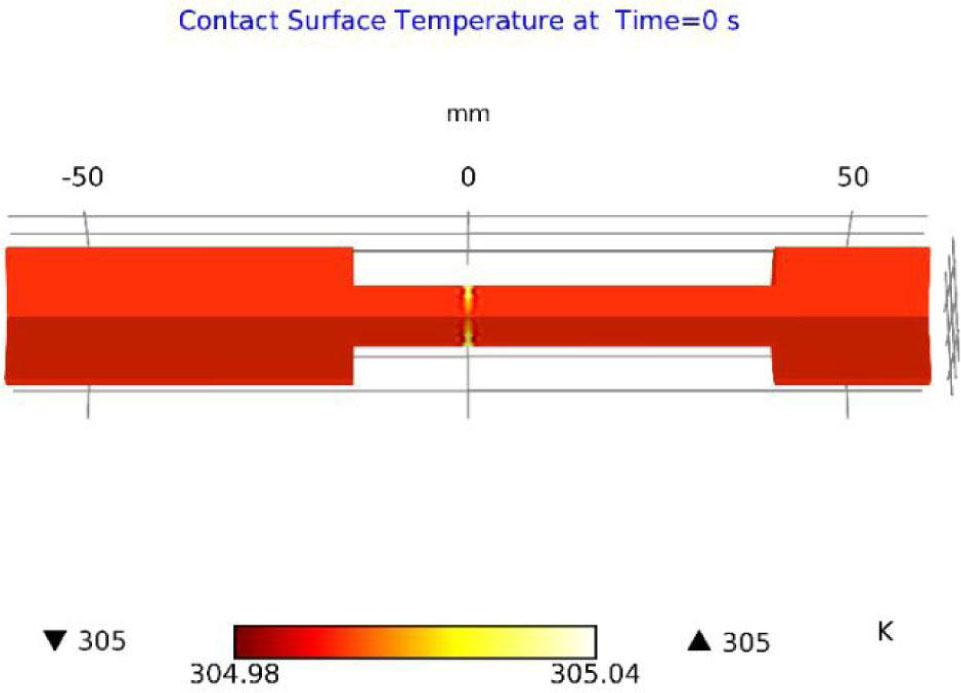Temperature profile at the contact zone at t = 0 s.

The first image (Figure 15) shows the two parts’ temperature profile before any rotation occurs and before any welding commences (t = 0 s). As indicated in that specific image, the two parts’ temperature is uniform and at the same temperature as the ambient temperature recorded during the actual welding experiments.

The images that show the temperature profile across the contact surfaces at the time steps: 1, 5, 10, and 11 s were zoomed-in purposely to visualize the temperature profile closely and monitor the progression of the temperature profiles and perhaps the welding zones. At t = 1 s, as shown in Figure 16, the maximum temperature increases to 348 K and is concentrated at the edges of the contact zone. The temperature at the center of the two parts is lower (about 320 K), and the spread of temperature is small. This outcome agrees with the heat generation model that predicts the increase of heat generation with radial distance according to Equations 8 and 9.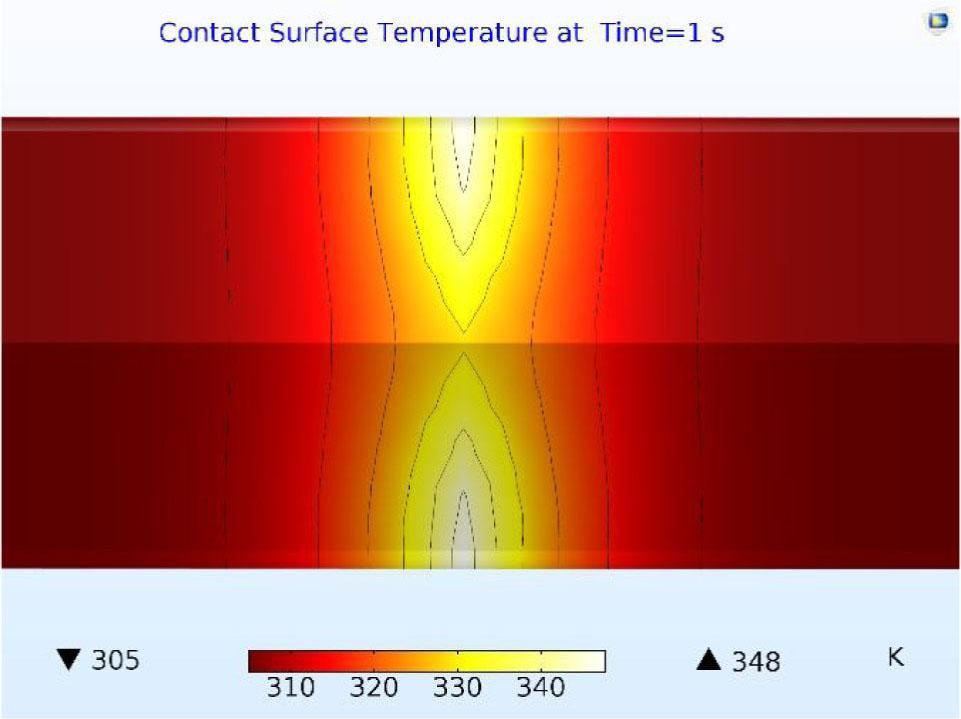Temperature profile at the contact zone at t = 1 s.

At t = 5 s, as seen in Figure 17, the temperature profile spreads more, and the maximum temperature reaches 387 K. This spread is indicated as bright white-small areas at the edges of the contact surfaces. It can also be noticed that there is an increased yellow area (360 < T < 390 K) around the contact surface of the parts to be welded. Another noticeable feature is that the maximum temperature area (bright white) that starts from the edges of the parts do not reach the center of the parts between zero and 5 s.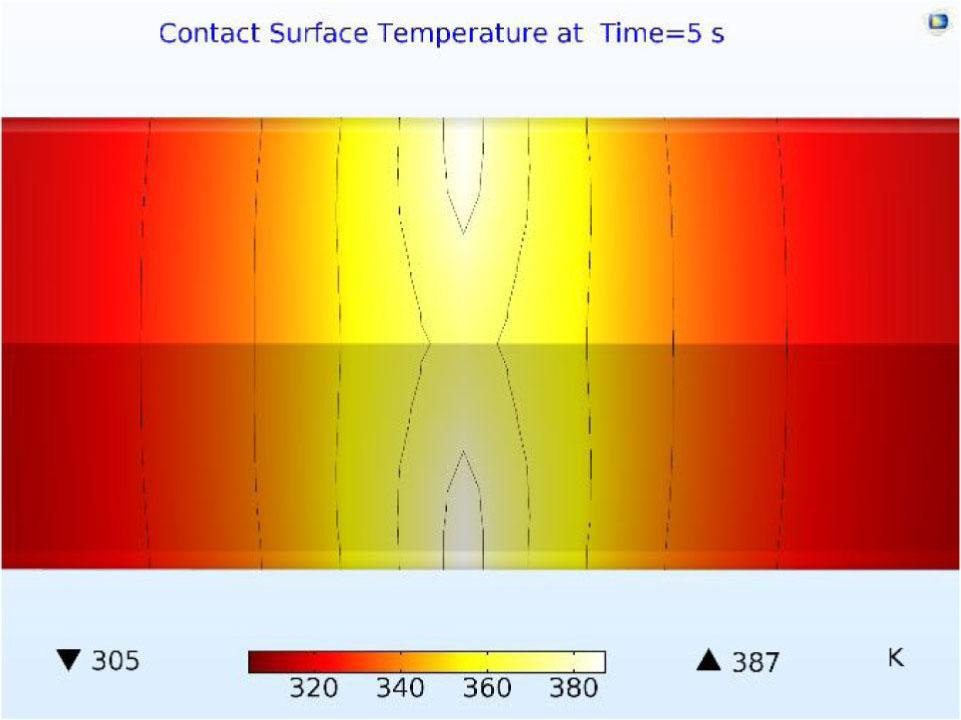Temperature profile at the contact zone at t = 5 s.

Figure 18 shows the temperature profile at the contact zones at 10 and 11 s, respectively. The profile at t = 10 s marks when rotation is stopped, and the profile at t = 11 s marks the time step immediately after that. At t = 10 s, as shown in Figure 18 (upper profile), the maximum temperature reaches 410 K. The high-temperature areas become broader and more pronounced compared to the time step of 5 s shown in Figure 17. Immediately after stopping the rotation (at t = 11 s), the maximum temperature falls back to 383 K, as shown in the lower profile in Figure 18. However, three distinct temperature areas become very clear and almost uniformly distributed. These are a very bright area at the center of the contact zone with a temperature of 383 K, a yellow area between 350 and 370 K surrounding the very bright area, and a colder orange area between 340 and 350 K on the outer edges of the contact zone. The profile at t = 11 s adds to the model's validity since the maximum temperature area changes from being at the edge due to the heat generated by rotation and friction to being concentrated around the center and more uniform.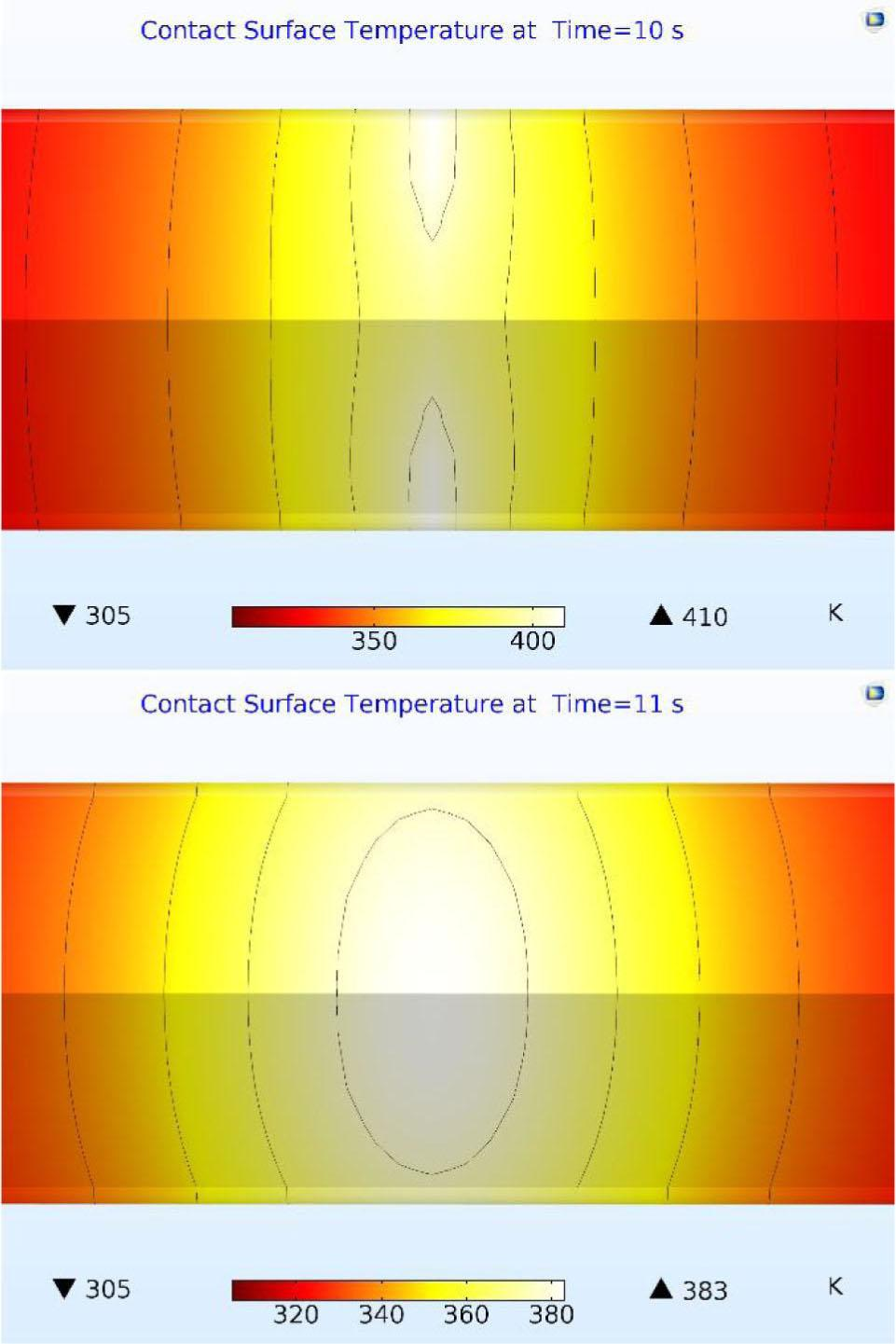The temperature profile at the contact zone at t = 10 and 11 s.
Discussion

As indicated in the previous section, experimental results show excellent agreement with the simulation model proposed. In general, the experimental method of measuring the applied temperature shows a new phenomenon not observed or maybe neglected in previous studies. That is the difference between the measured and modeled temperature profiles at the beginning of the welding cycle. This difference lasted between 2 and 3 seconds before providing readings similar to those presented by the model. As shown in Figure 11, this phenomenon occurs between 3 and 6 s, where the experimental curve exceeds the model curve. Afterward, the experimental curve increases, corresponding to the model's curve up to the maximum welding temperature.

This behavior is due to the displacement of the measuring point of the temperature as a function of time. Indeed, when the flash formation is triggered, the measuring point moves radially with the flash. As this point moves away from the contacting surfaces, the temperature drops slightly, then gradually rises to join the model. It was noted that the temperature's radial distribution is generally lower than its distribution over the entire length of the piece. The delay of the 3 s observed for the measuring point to reach the model's temperature is equal to the heat transfer rate setting time. Some experiments further investigated this phenomenon for friction times equal to 4, 5, 6, 9, and 10 s. Comparing the welded parts at different friction times showed that the flash formation passes through a critical point. This point consists of the flash evolution as a function of time, which translates, radially, to the movement of the temperature's measuring point. The flash occurs at specific time steps, and as the welding time increases, the flash size increases. This relevant result shows that the welding time is well defined and that the optimization of this time can improve the quality of joining such materials by CDFW.

The temperature profile and the three distinct areas of the model at t = 11 s, as shown in Figure 18, might be related to the welding zones that are usually observed in actual welding. The very bright area is probably the heat-affected zone of the welding. The yellow region may be an extension of the HAZ or perhaps another welding zone, such as a thermodynamically affected zone (TMAZ). The orange area could be another welding zone or a part of the base metal that did not undergo much heat or deformation. The width of the HAZ and the TMAZ can be a crucial determining factor for the welding quality. Microscopic analysis and mechanical tests of real welded joints must examine and verify the welding zones and any effects on the welded joints’ strength.

The numerical simulation of the two parts’ temperature distribution shows that this evolution follows the friction heat generation model. The temperature is more critical on the edge of the contact zone and decreases towards the center. This simulation provides a clear idea of the area affected by the temperature and identifies the more likely regions to be welded. In conclusion, the model chosen accurately reproduces the phenomenon of CDFW.

Conclusion

The use and development of continuous drive friction welding processes, used in various industrial applications, is an innovative and reliable alternative for assembling shafts, tubes, and many other geometries. A comprehensive approach has been developed in this field and consisted of constructing a CDFW model using the finite element method. The coupling of the process's thermal and mechanical aspects was considered during the COMSOL Multiphysics® simulation. The results found are very encouraging and offer an extensive understanding of the CDFW of Al6061. The adopted model has made it possible to build a modeling and simulation platform that gives rise to essential perspectives for testing the welding of other similar and dissimilar materials. The following conclusions can be drawn from this research effort:

Implementing a phase-change model and incorporating material thermal properties as functions of temperature can enhance the model results.

The excellent agreement between the experimental and model temperature profiles, especially regarding the maximum welding temperature.

Discrepancies between experimental and modeled temperature profiles before the maximum temperature are attributed to the welding flash formation mechanism due to the change of the measuring point of the temperature as a function of time as the flash formation is triggered and continued. In general, any effort that measures the experimental temperature at the edge of the contacting surfaces is expected to experience the same phenomenon.

Three-dimensional temperature profiles at different time intervals can point to the formation and evolution of HAZ of the welding. Such may shed some light on obtaining better quality welds.

#### Al6061 material properties for phase-change simulation. 

Property Value
Ttrans [K] 925.15
δT [K] 50
Δhf [kJ/kg] 380
ρsolid [kg/m3] 2705
ρliquid [kg/m3] 2415
Cp,solid [J/kg·K] 870
Cp,liquid [J/kg·K] 1170
κsolidus [W/m·K] 66.5
κliquid [W/m·K] 90

#### CDFW process parameters.

Process Parameter Value / range
Rotational Speed (rpm) 2000
Friction Pressure (MPa) 21
Friction time (s) 4, 5, 6, 9, 10, 12

#### FEM boundary conditions.

Model Feature Boundary Condition
Solid Mechanics The central axis of the parts Axial Symmetry
The outer surfaces of the parts Free to deform
The Entire model Zero initial displacement
The rotating part Rigid motion suppression
The end of the fixed part Axial load

Heat Transfer T = 305 K Initial temperature
The central axis of the parts Axial symmetry
The outer surfaces of the parts Heat flux according to q0 = h · (Text − T)

Welding Parameters Rotation 0 s to tf = rotation speed, otherwise zero
Pressure 0 s to tf = applied pressure, otherwise zero

#### Chemical composition in weight percent of Al6061.

Element Si Fe Cu Mn Mg Zn Cr Ti Al
Wt% 0.75 0.5 0.5 0.15 0.9 0.03 0.03 0.05 Rest

Sahin M. Joining of aluminium and copper materials with friction welding, Int J Adv Manuf Technol. 2010;49(5–8):527–34. https://doi.org/10.1007/s00170-009-2443-7. SahinM Joining of aluminium and copper materials with friction welding Int J Adv Manuf Technol 2010 49 5–8 527 34 https://doi.org/10.1007/s00170-009-2443-7. 10.1007/s00170-009-2443-7 Search in Google Scholar

Yilbas BS, Sahin AZ, Coban A, Abdul Aleem BJ. Investigation into the properties of friction-welded aluminium bars, J Mater Process Technol. 1995;54(1–4):76–81. https://doi.org/10.1016/0924-0136(95)01923-5. YilbasBS SahinAZ CobanA Abdul AleemBJ Investigation into the properties of friction-welded aluminium bars J Mater Process Technol 1995 54 1–4 76 81 https://doi.org/10.1016/0924-0136(95)01923-5. 10.1016/0924-0136(95)01923-5 Search in Google Scholar

Yang YC, Chen WL, Lee HL. A Nonlinear Inverse Problem in Estimating the Heat Generation in Rotary Friction Welding, Numer Heat Transf A. 2011;59(2):130–49. https://doi.org/10.1080/10407782.2011.540965. YangYC ChenWL LeeHL A Nonlinear Inverse Problem in Estimating the Heat Generation in Rotary Friction Welding Numer Heat Transf A 2011 59 2 130 49 https://doi.org/10.1080/10407782.2011.540965. 10.1080/10407782.2011.540965 Search in Google Scholar

Schmicker D, Persson P, Strackeljan J. Implicit Geometry Meshing for the simulation of Rotary Friction Welding, J Comput Phys. 2014;270:478–89. https://doi.org/10.1016/j.jcp.2014.04.014. SchmickerD PerssonP StrackeljanJ Implicit Geometry Meshing for the simulation of Rotary Friction Welding J Comput Phys 2014 270 478 89 https://doi.org/10.1016/j.jcp.2014.04.014. 10.1016/j.jcp.2014.04.014 Search in Google Scholar

Li W, Wang F. Modeling of continuous drive friction Welding of mild steel, Mater Sci Eng A. 2011;528(18):5921–6. https://doi.org/10.1016/j.msea.2011.04.001. LiW WangF Modeling of continuous drive friction Welding of mild steel Mater Sci Eng A 2011 528 18 5921 6 https://doi.org/10.1016/j.msea.2011.04.001. 10.1016/j.msea.2011.04.001 Search in Google Scholar

Kalsi NS, Sharma VS. A statistical analysis of rotary friction welding of steel with varying carbon in work-pieces, Int J Adv Manuf Technol. 2011;57(9–12):957–67. https://doi.org/10.1007/s00170-011-3361-z. KalsiNS SharmaVS A statistical analysis of rotary friction welding of steel with varying carbon in work-pieces Int J Adv Manuf Technol 2011 57 9–12 957 67 https://doi.org/10.1007/s00170-011-3361-z. 10.1007/s00170-011-3361-z Search in Google Scholar

Nguyen TC, Weckman DC. A thermal and microstructure evolution model of direct-drive friction welding of plain carbon steel, Metall Mater Trans, B, Process Metall Mater Proc Sci. 2006;37(2):275–92. https://doi.org/10.1007/BF02693157. NguyenTC WeckmanDC A thermal and microstructure evolution model of direct-drive friction welding of plain carbon steel Metall Mater Trans, B, Process Metall Mater Proc Sci 2006 37 2 275 92 https://doi.org/10.1007/BF02693157. 10.1007/BF02693157 Search in Google Scholar

Maalekian M, Kozeschnik E, Brantner HP, Cerjak H. Comparative analysis of heat generation in friction welding of steel bars, Acta Mater. 2008;56(12):2843–55. https://doi.org/10.1016/j.actamat.2008.02.016. MaalekianM KozeschnikE BrantnerHP CerjakH Comparative analysis of heat generation in friction welding of steel bars Acta Mater 2008 56 12 2843 55 https://doi.org/10.1016/j.actamat.2008.02.016. 10.1016/j.actamat.2008.02.016 Search in Google Scholar

Maalekian M, Cerjak H. Thermal-Phase Transformation Modelling and Neural Network Analysis of Friction Welding of Non-Circular Eutectoid Steel Components, Welding in the World 53 (2009) R44–R51. https://doi.org/10.1007/BF03266702. MaalekianM CerjakH Thermal-Phase Transformation Modelling and Neural Network Analysis of Friction Welding of Non-Circular Eutectoid Steel Components Welding in the World 53 2009 R44 R51 https://doi.org/10.1007/BF03266702. 10.1007/BF03266702 Search in Google Scholar

Can A., Sahin M., and Kucuk M. Modeling of Friction Welding, in: International Science Conference, 2010, II:135–142. CanA. SahinM. KucukM Modeling of Friction Welding in: International Science Conference 2010 II 135 142 Search in Google Scholar

Maalekian M. Friction welding critical assessment of literature, Sci Technol Weld Join. 2007;12(8):738–59. https://doi.org/10.1179/174329307X249333. MaalekianM Friction welding critical assessment of literature Sci Technol Weld Join 2007 12 8 738 59 https://doi.org/10.1179/174329307X249333. 10.1179/174329307X249333 Search in Google Scholar

Özdemir N. Investigation of the mechanical properties of friction-welded joints between AISI 304L and AISI 4340 steel as a function rotational speed, Mater Lett. 2005;59(19–20):2504–9. https://doi.org/10.1016/j.matlet.2005.03.034. ÖzdemirN Investigation of the mechanical properties of friction-welded joints between AISI 304L and AISI 4340 steel as a function rotational speed Mater Lett 2005 59 19–20 2504 9 https://doi.org/10.1016/j.matlet.2005.03.034. 10.1016/j.matlet.2005.03.034 Search in Google Scholar

Özdemir N., Sarsılmaz F., Hasçalık A. Effect of rotational speed on the interface properties of friction-welded AISI 304L to 4340 steel, Mater Des. 2007;28(1):301–7. https://doi.org/10.1016/j.matdes.2005.06.011. ÖzdemirN. SarsılmazF. HasçalıkA Effect of rotational speed on the interface properties of friction-welded AISI 304L to 4340 steel Mater Des 2007 28 1 301 7 https://doi.org/10.1016/j.matdes.2005.06.011. 10.1016/j.matdes.2005.06.011 Search in Google Scholar

Bouarroudj E, Chikh S, Abdi S, Miroud D. Thermal analysis during a rotational friction welding, Appl Therm Eng. 2017;110:1543–53. https://doi.org/10.1016/j.applthermaleng.2016.09.067. BouarroudjE ChikhS AbdiS MiroudD Thermal analysis during a rotational friction welding Appl Therm Eng 2017 110 1543 53 https://doi.org/10.1016/j.applthermaleng.2016.09.067. 10.1016/j.applthermaleng.2016.09.067 Search in Google Scholar

Dawood A, Butt S, Hussain G, Siddiqui M, Maqsood A, Zhang F. Thermal Model of Rotary Friction Welding for Similar and Dissimilar Metals, Metals (Basel) 2017;7(6):224. https://doi.org/10.3390/met7060224. DawoodA ButtS HussainG SiddiquiM MaqsoodA ZhangF Thermal Model of Rotary Friction Welding for Similar and Dissimilar Metals Metals (Basel) 2017 7 6 224 https://doi.org/10.3390/met7060224. 10.3390/met7060224 Search in Google Scholar

Juan J. Valencia and Peter N. Quested Thermophysical Properties, in: ASM Handbook, Volume 15. ASM International; 2008. pp. 468–81. JuanJ Valencia and Peter N. Quested Thermophysical Properties in: ASM Handbook 15 ASM International 2008 468 81 10.31399/asm.hb.v15.a0005240 Search in Google Scholar

Brandt R, Neuer G., Electrical Resistivity and Thermal Conductivity of Pure Aluminum and Aluminum Alloys up to and above the Melting Temperature, Int J Thermophys. 2007;28(5):1429–46. https://doi.org/10.1007/s10765-006-0144-0. BrandtR NeuerG Electrical Resistivity and Thermal Conductivity of Pure Aluminum and Aluminum Alloys up to and above the Melting Temperature Int J Thermophys 2007 28 5 1429 46 https://doi.org/10.1007/s10765-006-0144-0. 10.1007/s10765-006-0144-0 Search in Google Scholar

Leitner M, Leitner T, Schmon A, Aziz K, Pottlacher G. Thermophysical Properties of Liquid Aluminum, Metal-lurgical and Materials Transactions A. https://doi.org/10.1007/s11661-017-4053-6. LeitnerM LeitnerT SchmonA AzizK PottlacherG Thermophysical Properties of Liquid Aluminum Metal-lurgical and Materials Transactions A https://doi.org/10.1007/s11661-017-4053-6. 10.1007/s11661-017-4053-6 Search in Google Scholar

• #### Growth, spectral, optical, dielectric and third order nonlinear studies on 2-amino 4-methylpyridinium salicylate single crystals

Recommended articles from Trend MD